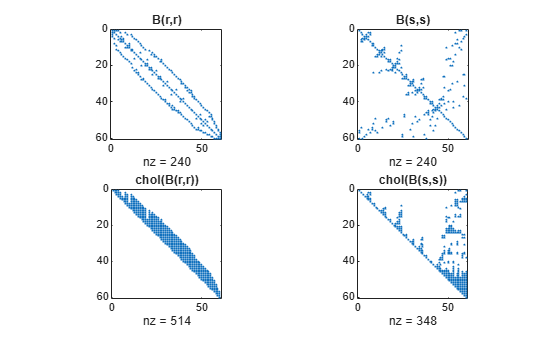# symamd

Symmetric approximate minimum degree permutation

## Syntax

```p = symamd(S) p = symamd(S,knobs) [p,stats] = symamd(...) ```

## Description

`p = symamd(S)` for a symmetric positive definite matrix `S`, returns the permutation vector `p` such that `S(p,p)` tends to have a sparser Cholesky factor than `S`. To find the ordering for `S`, `symamd` constructs a matrix `M` such that ```spones(M'*M) = spones (S)```, and then computes `p = colamd(M)`. The `symamd` function may also work well for symmetric indefinite matrices.

`S` must be square; only the strictly lower triangular part is referenced.

`p = symamd(S,knobs)` where `knobs` is a scalar. If `S` is `n`-by-`n`, rows and columns with more than `knobs*n` entries are removed prior to ordering, and ordered last in the output permutation `p`. If the `knobs` parameter is not present, then `knobs = spparms('wh_frac')`.

`[p,stats] = symamd(...)` produces the optional vector `stats` that provides data about the ordering and the validity of the matrix `S`.

 `stats(1)` Number of dense or empty rows ignored by `symamd` `stats(2)` Number of dense or empty columns ignored by `symamd` `stats(3)` Number of garbage collections performed on the internal data structure used by `symamd` (roughly of size `8.4*nnz(tril(S,-1)) + 9n` integers) `stats(4)` `0` if the matrix is valid, or `1` if invalid `stats(5)` Rightmost column index that is unsorted or contains duplicate entries, or `0` if no such column exists `stats(6)` Last seen duplicate or out-of-order row index in the column index given by `stats(5)`, or `0` if no such row index exists `stats(7)` Number of duplicate and out-of-order row indices

Although, MATLAB® built-in functions generate valid sparse matrices, a user may construct an invalid sparse matrix using the MATLAB C or Fortran APIs and pass it to `symamd`. For this reason, `symamd` verifies that `S` is valid:

• If a row index appears two or more times in the same column, `symamd` ignores the duplicate entries, continues processing, and provides information about the duplicate entries in `stats(4:7)`.

• If row indices in a column are out of order, `symamd` sorts each column of its internal copy of the matrix `S` (but does not repair the input matrix `S`), continues processing, and provides information about the out-of-order entries in `stats(4:7)`.

• If `S` is invalid in any other way, `symamd` cannot continue. It prints an error message, and returns no output arguments (`p` or `stats`).

The ordering is followed by a symmetric elimination tree post-ordering.

## Examples

collapse all

Here is a comparison of reverse Cuthill-McKee and minimum degree on the Bucky ball example mentioned in the `symrcm` reference page.

```B = bucky+4*speye(60); r = symrcm(B); p = symamd(B); R = B(r,r); S = B(p,p); subplot(2,2,1), spy(R,4), title('B(r,r)') subplot(2,2,2), spy(S,4), title('B(s,s)') subplot(2,2,3), spy(chol(R),4), title('chol(B(r,r))') subplot(2,2,4), spy(chol(S),4), title('chol(B(s,s))')```Even though this is a very small problem, the behavior of both orderings is typical. RCM produces a matrix with a narrow bandwidth which fills in almost completely during the Cholesky factorization. Minimum degree produces a structure with large blocks of contiguous zeros which do not fill in during the factorization. Consequently, the minimum degree ordering requires less time and storage for the factorization.

## References

The authors of the code for `symamd` are Stefan I. Larimore and Timothy A. Davis (`davis@cise.ufl.edu`), University of Florida. The algorithm was developed in collaboration with John Gilbert, Xerox PARC, and Esmond Ng, Oak Ridge National Laboratory. Sparse Matrix Algorithms Research at the University of Florida: `https://www.cise.ufl.edu/research/sparse/`